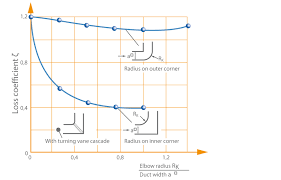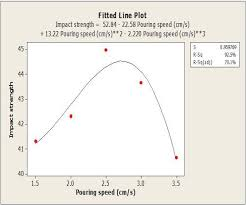## How to Calculate and Solve for Total Cross-Sectional Areas of Gates | Design of Gating System by a Hydraulic MethodThe image above represents total cross-sectional areas of gates.

To compute for total cross-sectional areas of gates, four essential parameters are needed and these parameters are mass of the casting (G), density of melt (ρ), pouring time (t) and pouring speed (v).

The formula for calculating total cross-sectional areas of gates:

Fg = G / ρtv

Where:

Fg = Total Cross-Sectional Area of Gates
G = Mass of the Casting
ρ = Density of Melt
t = Pouring Time
v = Pouring Speed

Let’s solve an example;
Find the total cross-sectional areas of gates when mass of the casting is 12, density of melt is 16, pouring time is 5 and pouring speed is 25.

This implies that;

G = Mass of the Casting = 12
ρ = Density of Melt = 16
t = Pouring Time = 5
v = Pouring Speed = 25

Fg = G / ρtv
Fg = 12 / 16 x 5 x 25
Fg = 12 / 2000
Fg = 0.006

Therefore, the total cross-sectional areas of gates is 0.006 m2.

Calculating the Mass of the Casting when the Total Cross-Sectional Areas of Gates, the Density of Melt, the Pouring Time and the Pouring Speed is Given.

G = Fg x ρtv

Where;

G = Mass of the Casting
Fg = Total Cross-Sectional Area of Gates
ρ = Density of Melt
t = Pouring Time
v = Pouring Speed

Let’s solve an example;
Find the mass of the casting when the total cross-sectional areas of gates is 32, the density of melt is 4, the pouring time is 10 and the pouring speed is 2.

This implies that;

Fg = Total Cross-Sectional Area of Gates = 32
ρ = Density of Melt = 4
t = Pouring Time  = 10
v = Pouring Speed = 2

G = Fg x ρtv
G = 32 x 4 x 10 x 2
G = 2560

Therefore, the mass of the Casting is 2560 m.

## How to Calculate and Solve for Pouring Speed | Design of Gating SystemThe image above represents pouring speed.

To compute for poring speed, three essential parameters are needed and these parameters are Co-efficient for friction (μ), Acceleration due to Gravity (g) and Rated head static (Hs).

The formula for calculating pouring speed:

v = μ√(2gHs)

Where:

v = Pouring Speed
μ = Co-efficient for Friction
g = Acceleration due to Gravity

Let’s solve an example;
Find the pouring speed when the co-efficient for friction is 14, acceleration due to gravity is 9 and rated static head is 16.

This implies that;

μ = Co-efficient for Friction = 14
g = Acceleration due to Gravity = 9
Hs = Rated Static Head = 16

v = μ√(2gHs)
v = 14 x √(2 x 9 x 16)
v = 14 x √(288)
v = 14 x 16.97
v = 237.58

Therefore, the pouring speed is 237.58 m/s.

Calculating the Co-efficient for Friction when the Pouring Speed, the Acceleration due to Gravity and the Rated Static Head is Given.

μ = v / √(2gHs)

Where;

μ = Co-efficient for Friction
v = Pouring Speed
g = Acceleration due to Gravity

Let’s solve an example;
Find the co-efficient for friction when the pouring speed is 24, the acceleration due to gravity is 9 and the rated static head is 11.

This implies that;

v = Pouring Speed = 24
g = Acceleration due to Gravity = 9
Hs = Rated Static Head = 11

μ = v / √(2gHs)
μ = 24 / √(2 x 9 x 11)
μ = 24 / √198
μ = 24 / 14.07
μ = 1.70

Therefore, the co-efficient for friction is 1.70.